# Geometry Toolbox

### Ideas for activities

Angles in a Triangle

Show that when you move the points of a triangle the angles always sum to 180o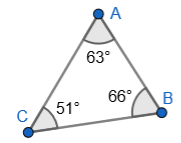Angle in a Semicircle

Show that the angle drawn in at the circumference from either ends of the diameter is always 90o.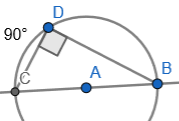Polygons

Make a poster showing the basic polygons and their names. For example

Triangle

Pentagon

Hexagon

Heptagon

Parallel Lines

Move intersecting parallel lines to show that alternate, vertically opposite and corresponding angles are always equal.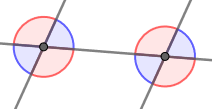Tangram

Accurately construct the perfect Tangram puzzle after first calculating the required lengths and angles.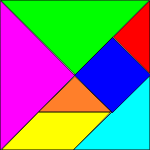Rotational Symmetry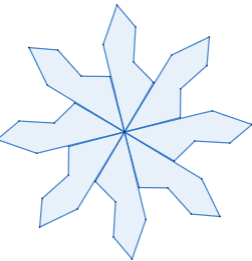Triangle Centres

Research different ways of finding the centre of a triangle. Investigate the conditions under which at least two of the following coincide:

Centroid

Circumcenter

Incenter

Orthocenter

Unit Circle

Show the relationship between sine and cosine in a dynamic unit circle.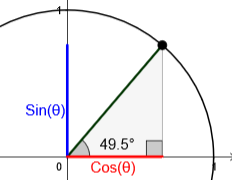Transformations

Find the transform tools then make dynamic diagram showing what each type of transformation looks like.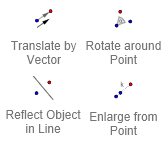### Related activities

#### Angle ChaseFind all of the angles on the geometrical diagrams. Use your knowledge of basic angle theorems.

Transum.org/go/?to=chase

#### Area Two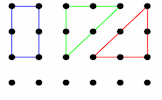How many different shapes with an area of 2 square units can you make by joining dots on this grid?

Transum.org/go/?to=area2

#### Polygon Angles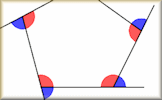A mixture of problems related to calculating the interior and exterior angles of polygons.

Transum.org/go/?to=polyang

#### Circle Theorems ExerciseShow that you understand and can apply the circle theorems with this self marking exercise.

Transum.org/go/?to=circleangles

Do you have any comments? It is always useful to receive feedback and helps make this free resource even more useful for those learning Mathematics anywhere in the world. Click here to enter your comments.For All: